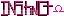-=+=- -=+=- -=+=- -=+=- -=+=- -=+=- -=+=- -=+=- -=+=- -=+=- -=+=- -=+=- -=+=- -=+=- -=+=- -=+=- -=+=- -=+=- -=+=- -=+=- -=+=- -=+=- -=+=- -=+=- -=+=- -=+=- -=+=- -=+=- -=+=- -=+=- (c) WidthPadding Industries 1987 0|24|0 -=+=- -=+=- -=+=- -=+=- -=+=- -=+=- -=+=- -=+=- -=+=- -=+=- -=+=- -=+=- -=+=- -=+=- -=+=- -=+=- -=+=- -=+=- -=+=- -=+=- -=+=- -=+=- -=+=- -=+=- -=+=- -=+=- -=+=- -=+=- -=+=- -=+=-
SoCoder -> Blogs Home -> Blogs

magicmanCreated : 16 February 2008

Today my science club from school(that i started) competed in the annual science Olympiad regional tournament. I thought we were going to do horribly seeing as how the only preparation we took was to build the things that required building, we didnt even really do that! the robot didnt work at all, and the electric vehicle didnt do what it was suppose to, and nor did the plane fly. However, despite the poorest of all poor preparations for the event, we some how managed to get first place and are now going to state! Its amazing that we could possibly pull such a thing off, especially since we formed the team all but 2 or 3 days ago.

Saturday, 16 February 2008, 17:10
Schererererthat's really cool, congradulations! Maybe at state you'll be more prepared, hahaSaturday, 16 February 2008, 17:36
power mouseycongratulations.Sunday, 17 February 2008, 13:09
HoboBenCool, well done!Sunday, 17 February 2008, 15:02
JayenkaiHow come I never got to anything cool like that at school!?

Sounds like fun. Well done.
Good luck at the State tournament.